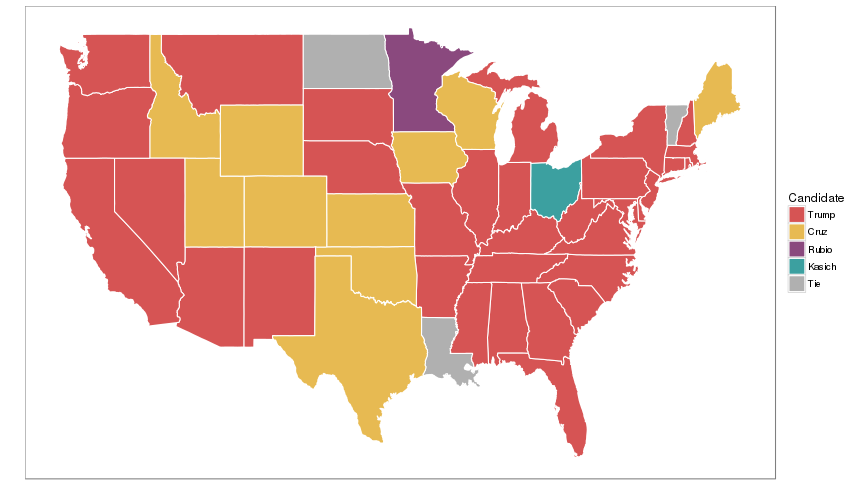# Presidential Candidate Delegate Count

## Purpose

I mostly made this because while the New York Times' delegate count (the source of this data) is very good at providing maps, they generally show a bit too much information for certain things. In some cases their mapping cannot show you exactly who won a state because the mapping is done by county. They do provide mapping based on how much a state favored a certain candidate but they don't have all candidates on the same map by state, only by county.

What people really want is much simpler data than this: who won each state. It's a misleading map at times which is why the New York Times probably doesn't publish it. If Clinton won against Sanders by 28 to 27 (Kentucky), the state in question looks like Clinton won it outright when in fact it was a close race. Also, state lines can be really arbitrary, and the level of detail the New York Times provides is quite a bit more useful. Think about St. Louis which straddles Illinois and Missouri, yet can impact the outcome of multiple states.

So these are the maps that people want, and even though I know myself they're misleading, even I want to see them. If I want to see something, I see it. If I want to do something, I do it. So I did it, and I see it.

## Libraries

``````library(ggplot2)
library(reshape2)
``````

## Style Functions

``````colors <- data.frame(
color=c(
"#d65454", "#e7ba52",
"#8a497e", "#3ca0a0",
"#4a8fd3", "#8ecc64",
"#b0b0b0"
),
candidate=c(
"Trump", "Cruz",
"Rubio", "Kasich",
"Clinton", "Sanders",
"Tie"
)
)
color_convert <- function(data){
sapply(data, function(x){
colors\$color[
colors\$candidate == as.character(x)
]
})
}
``````
``````plot_style <- function(g){
g + theme_bw() + theme(
panel.grid=element_blank(),
axis.text=element_blank(),
axis.ticks=element_blank()
) + scale_color_identity(
"Candidate",
labels=colors\$candidate,
breaks=colors\$color,
guide="legend"
)  + scale_fill_identity(
"Candidate",
labels=colors\$candidate,
breaks=colors\$color,
guide="legend"
) + xlab("") + ylab("")
}
``````

This data is taken from here: http://www.nytimes.com/interactive/2016/us/elections/primary-calendar-and-results.html

``````del <- read.csv("delegates.csv")
states_map <- map_data("state")
``````
``````##
##  # maps v3.1: updated 'world': all lakes moved to separate new #
##  # 'lakes' database. Type '?world' or 'news(package="maps")'.  #
``````
``````del\$Date.Democratic <- as.POSIXlt(del\$Date.Democratic)
del\$Date.Republican <- as.POSIXlt(del\$Date.Republican)
``````

## Generate Winners by State

``````winners <- function(cand){
del_subset <- del[,c("State",cand)]
winz <- data.frame(State=del_subset\$State, Winner=NA)
for(i in 1:nrow(del_subset)){
result <- seq(1,length(cand))[
del_subset[i,cand] == max(del_subset[i,cand])
]
if(length(result) == 1){
winz\$Winner[i] <- cand[result]
}
}
winz\$Winner[is.na(winz\$Winner)] <- "Tie"
names(winz) <- c("state", "Winner")
winz\$state <- tolower(as.character(winz\$state))
winz\$Winner <- factor(winz\$Winner)
winz
}
``````
``````win_dem <- winners(c("Clinton","Sanders"))
win_rep <- winners(c("Trump","Cruz","Rubio","Kasich"))
``````
``````states <- as.character(levels(factor(states_map\$region)))
win_dem <- win_dem[win_dem\$state %in% states,]
win_rep <- win_rep[win_rep\$state %in% states,]
``````

## Generate Delegates by Time

``````timeline_datagen <- function(date_field, cands){
del_subset <- del[
!is.na(del[[date_field]]) & !is.na(del[[cands]]),
c(date_field, cands)
]
del_subset <- del_subset[order(del_subset[[date_field]]),]

del_date <- del_subset[[date_field]]
del_subset[[date_field]] <- NULL
del_subset <- melt(del_subset, NULL)
del_subset\$Date <- as.numeric(del_date)
del_agg <- aggregate(
value ~ variable + Date,
data=del_subset, FUN=sum
)
del_agg <- del_agg[order(del_agg\$variable, del_agg\$Date),]
names(del_agg) <- c("Candidate", "Date", "Sum")
del_agg
}
timeline_cumsum <- function(del_agg){
del_cumsum <- tapply(del_agg\$Sum, del_agg\$Candidate, FUN=cumsum)
del_agg\$Sum <- unlist(del_cumsum)
del_agg
}
``````
``````time_dem <- timeline_datagen("Date.Democratic", c("Clinton","Sanders"))
time_rep <- timeline_datagen("Date.Republican", c("Trump","Cruz","Rubio","Kasich"))
cumtime_dem <- timeline_cumsum(time_dem)
cumtime_rep <- timeline_cumsum(time_rep)
``````

## Plotting Functions

``````plot_timeline <- function(data){
data\$Candidate <- color_convert(data\$Candidate)
g <- ggplot(data, aes(y=Sum, x=Date, color=Candidate))
g <- g + geom_line()
plot_style(g)
}
``````
``````cand_map <- function(data){
data\$Winner <- color_convert(data\$Winner)
g <- ggplot(data, aes(map_id=state))
g <- g + geom_map(aes(fill=Winner), color="white", map=states_map)
g <- g + expand_limits(x=states_map\$long, y=states_map\$lat)
plot_style(g)
}
``````

## Plots

``````plot_timeline(time_dem)
``````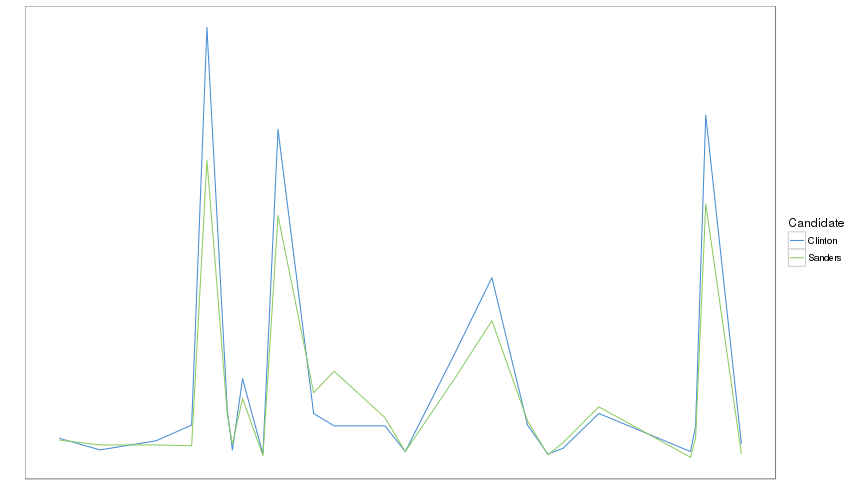``````plot_timeline(time_rep)
``````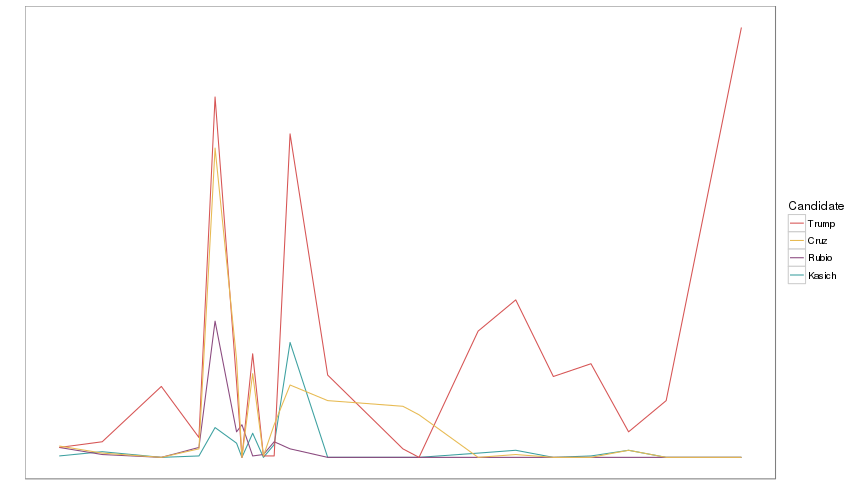``````plot_timeline(cumtime_dem)
``````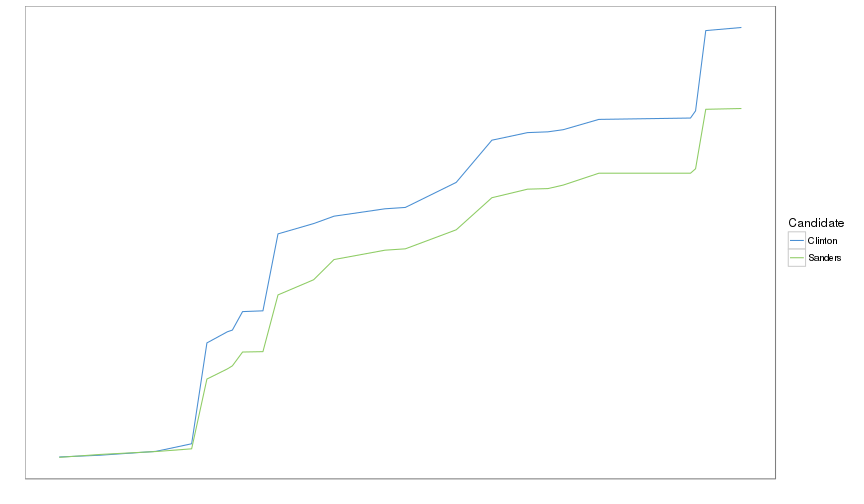``````plot_timeline(cumtime_rep)
``````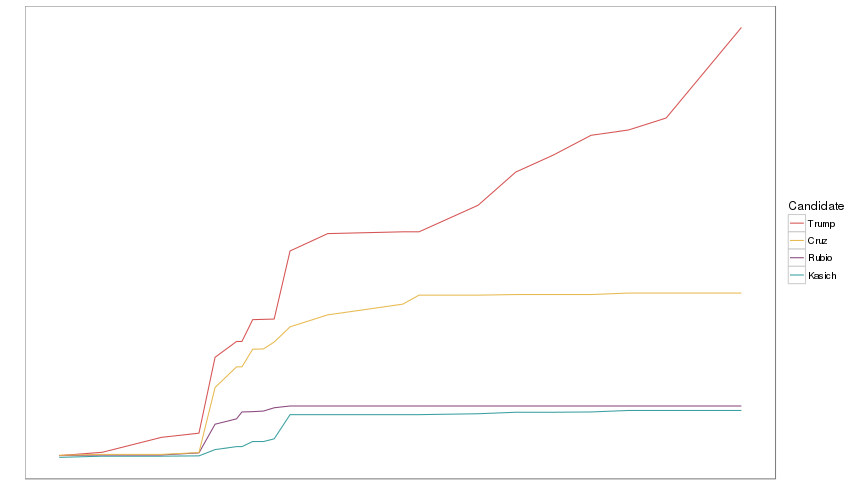``````cand_map(win_dem)
``````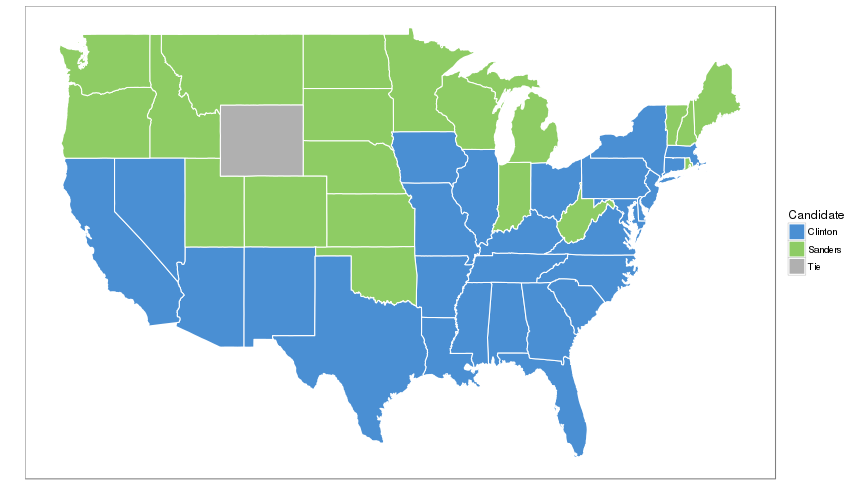``````cand_map(win_rep)
``````